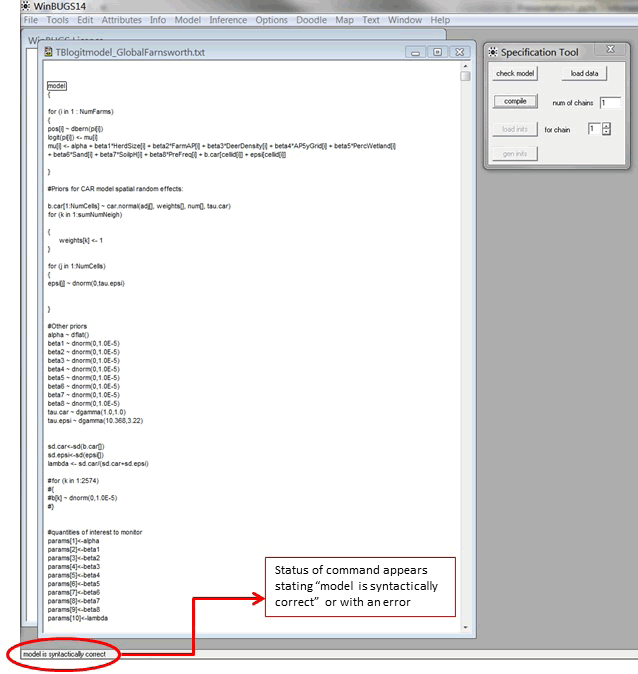The Check Model component determines if the model structure is presented properly for the program to run. If the model structure is appropriate, the bottom left corner of the screen will read model is syntactically correct (Fig. 9.2).

model
{
for (i in 1 : NumFarms)
{
pos[i] ~ dbern(pi[i])
logit(pi[i]) <- mu[i]
mu[i] <- alpha + beta1*DeerDensity[i] + beta2*AP5yGrid[i] + beta3*PercWetland[i]
+ beta4*Sand[i] + beta5*SoilpH[i] + beta6*PreFreq[i] + b.car[cellid[i]] + epsi[cellid[i]]
}Figure 9.2: Compiling the model structure in WinBUGS